# Math in Focus Grade 6 Chapter 6 Lesson 6.4 Answer Key Real-World Problems: Percent

Practice the problems of Math in Focus Grade 6 Workbook Answer Key Chapter 6 Lesson 6.4 Real-World Problems: Percent to score better marks in the exam.

## Math in Focus Grade 6 Course 1 A Chapter 6 Lesson 6.4 Answer Key Real-World Problems: Percent

### Math in Focus Grade 6 Chapter 6 Lesson 6.4 Guided Practice Answer Key

Solve.

Question 1.
Mr. Jefferson is making 80 cups of fruit punch for the grand opening of his bakery. He uses 52 cups of fruit juice and the rest is sparkling water.
a) What percent of the punch is fruit juice?
Method 1
Fraction of fruit juice in punch = $$\frac{?}{?}$$
Percent of fruit juice in punch = $$\frac{?}{?}$$ ×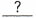%
=%
=% of the punch is fruit juice.

Method 2cups → 100%
1 cup → $$\frac{100}{?}$$%cups → ? × $$\frac{100}{?}$$ =%% of the punch is fruit juice.
65%
Explanation:
Method 1
Fraction of fruit juice in punch = $$\frac{52}{80}$$
Percent of fruit juice in punch = $$\frac{52}{80}$$ × 100%
= 65%
= 65% of the punch is fruit juice.

b) What percent of the punch is sparkling water?
100% –% =%% of the punch is sparkling water.
35%
Explanation:
100% – 65% = 35%
35% of the punch is sparkling water.

Solve. Check for reasonableness.

Question 2.
A laptop computer displayed at a shop costs $720. A sales tax of 7% will be added to the price. What is the total cost of the laptop computer? Method 1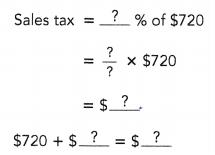The total cost of the laptop computer is$.

Method 2
Sales tax: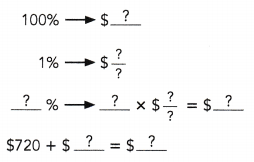The total cost of the laptop computer is $. Answer:$770.40
Explanation: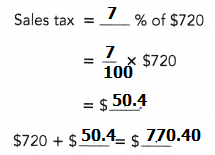Question 3.
Dave went to lunch with his friends. The food cost $78.50, and a sales tax of$4.71 was added to the cost of the meal. What was the sales tax rate?
The cost of the food is%.
$%$1 → $$\frac{?}{?}$$ %
$× $$\frac{?}{?}$$ % =% The sales tax rate was%. Answer: 6% Explanation: The cost of the food is$78.50 %.
$4.71 → 1%$1 → $$\frac{4.71}{78.50}$$ %
$100 → 100× $$\frac{471}{7850}$$ % = 0.06% The sales tax rate was 6%. Question 4. Mr. Diaz earns a 3% commission on all the furniture he sells. If he receives$2,880 in commission, what is the dollar amount of his sales?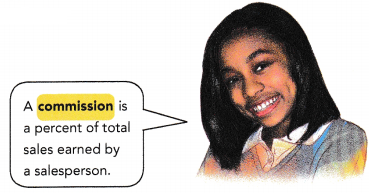% → $1% →$$$\frac{?}{?}$$ % = $% 100% → 100 ×$= $The dollar amount of his sales is$.
$96,000 Explanation: 3% →$2,880
1% → $$$\frac{2880}{3}$$ % =$960 %
100% → 100 × $960=$96,000
The dollar amount of his sales is $96,000. Solve. Question 5. A firm has$30,000 in a bank account at the beginning of the year. Interest will be paid at a rate of 2% at the end of the year. How much interest will the firm receive for the year?
Interest = imgg 1 % of $for 1 year = $$\frac{?}{?}$$ ×$× 1
= $The firm will receive$in interest for the year.
$600 Explanation: Interest = imgg 1 % of$30,000 for 1 year
= $$\frac{2}{100}$$ × $30,000 × 1 = 2 X 300 =$600

Question 6.
A company has $500,000 in a savings account. The interest is 4% per year. How much interest will it earn at the end of $$\frac{1}{2}$$ year? Interest = $$\frac{?}{100}$$ ×$× $$\frac{?}{?}$$
= $The company will earn$in interest at the end of $$\frac{1}{2}$$ year.
$10,000 Explanation: Interest = $$\frac{4}{100}$$ ×$500,000 × $$\frac{1}{2}$$
= 20,000 X $$\frac{1}{2}$$
= $10,000 The company will earn$10,000 in interest at the end of $$\frac{1}{2}$$ year.

### Math in Focus Course 1A Practice 6.4 Answer Key

Question 1.
Ellen had 25 hair clips. 7 of them were blue and the rest were purple.
a) What percent of the hair clips were blue?
28%
b) What percent of the hair clips were purple?
72%
Explanation:
7 of them were blue and the rest were purple.
Number of blue clips = 7
Number of purple clips = 125 – 7 = 18
percentage of blue clips = $$\frac{7}{25}$$ × 100 = 28%
percentage of purple clips = $$\frac{18}{25}$$ × 100 = 72%

Question 2.
Gabriel had $60. He spent$36 on a pair of shoes and the rest on a shirt.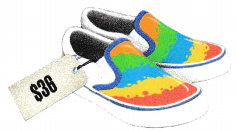a) What percent of the money did he spend on the pair of shoes?
60%
b) What percent of the money did he spend on the shirt?
40%
Explanation:
Gabriel had $60. He spent$36 on a pair of shoes and the rest on a shirt.
percent of the money did he spend on the pair of shoes
= $$\frac{36}{60}$$ × 100
= 60%
Total cost spent on shirt = 60 – 36 = $24 percent of the money did he spend on the shirt = $$\frac{24}{60}$$ × 100 = 40% Question 3. Ms. Pierce bought a camera that cost$450. In addition, she had to pay 4% u sales tax. How much did Ms. Pierce pay for the camera?
$468 Explanation: Ms. Pierce bought a camera that cost$450.
Total price of the camera = $$\frac{4}{100}$$ × 450 = $18 Total amount he paid = camera cost + tax =$450 + $18 =$468

Question 4.
Mr. Osmond bought a computer that cost $2,500. How much did he pay for the computer if the sales tax rate was 7%? Answer:$2,675
Explanation:
Mr. Osmond bought a computer that cost $2,500. Tax paid for the computer was 7%. Total amount he paid = $$\frac{7}{100}$$ × 2,500 =$175
Total amount he paid including tax = cost of computer + tax
= $3,500 +$175 = $2,675 Question 5. There were 320 girls and 180 boys at a playground. What percent of children were girls? Answer: 64% Explanation: There were 320 girls and 180 boys at a playground. Total boys and girls = 320 + 180 = 500 percent of children were girls = $$\frac{320}{500}$$ × 100 = 64% Question 6. Sally spent$36 and had $12 left. What percent of her money did she spend? Answer: 75% Explanation: Sally spent$36 and had $12 left. Total amount = spent + left =$36 + $12 =$48
percent of her money did she spend
= $$\frac{36}{48}$$ × 100 = 75%

Question 7.
An artist receives 20% royalty on the retail price of his recordings. If he receives $36,000 in royalties, what is the dollar amount of the retail price of his recordings? Answer:$18,000,000
Explanation:
An artist receives 20% royalty on the retail price of his recordings.
If he receives $36,000 in royalties, Total dollar amount of the retail price of his recordings $$\frac{20}{100}$$ of X = 36,000 100% of X = ? X = 36000 x $$\frac{100}{20}$$ x 100 X = 36,000 x 5 x 100 X = 18,000,000 Question 8. A club deposited$50,000 in a savings account at the beginning of the year. Interest will be paid at a rate of 3% at the end of the year. How much interest will the club receive for the year?
$1,500 Explanation: A club deposited$50,000 in a savings account at the beginning of the year.
Interest will be paid at a rate of 3% at the end of the year.
Total interest will the club receive for the year
= $$\frac{3}{100}$$ × 50,000 = 1,500

Question 9.
Company X has $128,000 in a savings account that pays 6% interest per year. How much interest will it earn at the end of $$\frac{1}{2}$$ year? Answer:$3,840
Explanation:
Company X has \$128,000 in a savings account that pays 6% interest per year.
= $$\frac{6}{100}$$ × 128,000 = 7,680
Total interest will it earn at the end of $$\frac{1}{2}$$ year
= $$\frac{1}{2}$$ × 7,680 = 3,840